# How to Remove Decimals in Excel & Google Sheets

This tutorial demonstrates how to remove decimals in Excel and Google Sheets.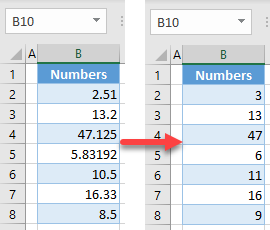## Remove Decimals Using Cell Formatting

Say you have the following dataset containing decimal numbers.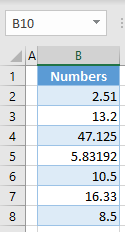To remove decimals by formatting, follow these steps:

1. Select the range with decimal numbers (B2:B8), right-click the selected area, and choose Format Cells…
You can, alternatively, go to the Home tab in the Ribbon, and click on the Format Number icon in the bottom-right corner of the Number group.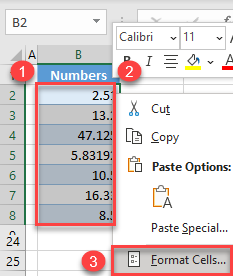1. In the Format Cells window, go to the Number category, set 0 Decimal places, and click OK.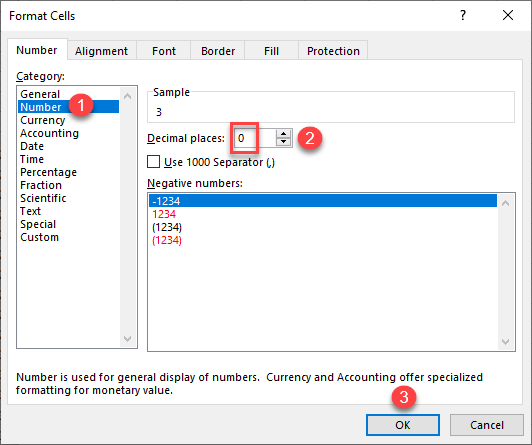As a result, all numbers are rounded to the nearest whole number.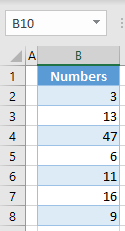## Remove Decimals Without Rounding

If you want just to remove decimals without rounding use the TRUNC Function.

1. Create one helper column, and in cell C2, enter the formula:
`=TRUNC(B2)`
1. Apply the formula through the last row with data (Row 8).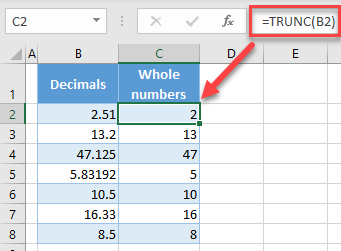As you can see, decimals are now truncated (not rounded) from the original number, and you got whole numbers without any of the decimal remainders.

## Round to Remove Decimals

You can also remove decimals and output rounded numbers. To achieve this, use the ROUND Function with zero decimal places.

1. In cell C2, enter the formula:
`=ROUND(B2,0)`
1. Apply the formula through the last row with data (Row 8).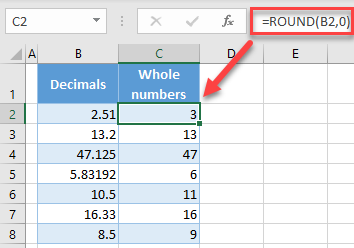Alternatively, you can use the INT (which rounds decimal to a whole number), ROUNDUP, or ROUNDDOWN Function.

## Remove Decimals Using Cell Formatting in Google Sheets

1. Select the range with decimal numbers (B2:B8), and in the Menu, go to Format > Number > Custom number format.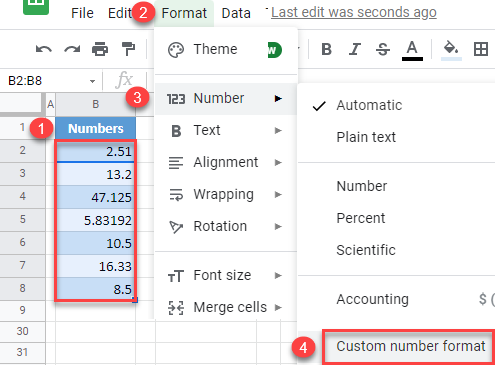1. In the Custom number formats window, enter 0 for whole numbers, and click OK.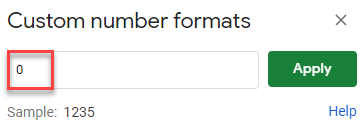As a result, all numbers are rounded to the nearest whole number.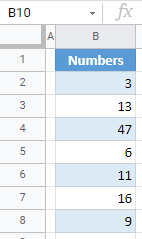Note: Similarly to Excel, you can also use functions:

• ROUND
• TRUNC – to remove decimals without rounding
• INT, ROUNDUP, ROUNDDOWN – to remove decimals and round a number